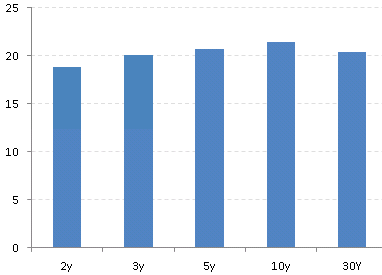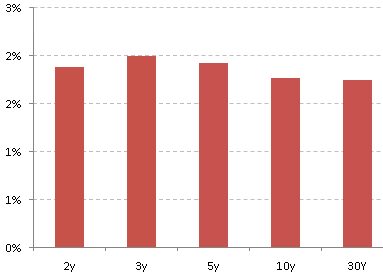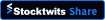Saturday, April 28, 2012

Absolute vs. relative volatility of rates

Risk measurement models often attempt to project the behavior of the yield curve based on historical rate movements. But the assumptions that go into such modeling could often produce misleading results. Here is why.

The chart below shows the recent historical volatility of yields along the US treasury curve. These are the standard deviations of weekly rate changes in basis points over the past 16 months (from 1/1/11 to today). The historical volatility curve shown here makes sense intuitively, with longer term yields being absolutely more volatile than the short term yields.Absolute weekly historical volatility of rates from 1/1/11 to 4/27/12 in basis points

But that's not the whole story. An alternative way of looking at rate volatility is on a relative basis. That would be the change in each rate as a proportion of the rate at the time. For example if the 10-year rate is 2% and it moves by 10 basis points, the relative move would be 5% (10bp/200bp). This model tells us that if the 10-year rate becomes 4%, the equivalent move would be 20bp (5% of 4%). As rates rise, so do the expected moves in basis points. The shape of the relative historical volatility is shown below and has the opposite slope of the absolute volatility. This is also fairly intuitive because even small rate moves at the short end produce large relative moves with rates being close to zero.Relative weekly historical volatility of rates from 1/1/11 to 4/27/12
(% of rate)

Which one is the correct approach to model movements in interest rates? Rate movements are not like stocks for example that can almost always be modeled using relative moves (lognormal distribution). But using absolute movements is not always correct either because a 10bp move in the 2-year rate in 2006 was routine but would be considered a huge and far less likely move now when short-term rates are near zero. The reality is somewhere in-between.

Also the shape of the rate volatility curve has not always been the same. The charts below show the same results for the 2006-2007 period (unfortunately the 1-year and the 7-year data was not available for that period). Not only is the absolute and the relative volatility far smaller than the current levels, but the volatility curves are both relatively flat (in part due to the flat or inverted yield curve during that period).Absolute weekly historical volatility of rates from 1/1/06 to 4/27/07 in basis pointsRelative weekly historical volatility of rates from 1/1/06 to 4/27/07
(% of rate)

So the next time your risk manager shows you a VAR number of a rate product portfolio, ask her which volatility and term structure assumptions as well as the historical periods were used. The answer could make all the difference.

SoberLook.comBookmark this post: